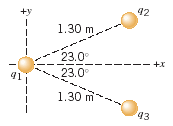# 42 +y 1.30 m 23.0 23.0 - +x 1.30 m 43

Question

The drawing shows three point charges fixed in place. The charge at the coordinate origin has a value of q1 = +7.37C; the other two have identical magnitudes, but opposite signs: q2 = -7.41C and q3 = +7.41C. (a) Determine the net force exerted on q1 by the other two charges. (b) If q1 had a mass of 1.50 g and it were free to move, what would be its acceleration?help_outlineImage Transcriptionclose42 +y 1.30 m 23.0 23.0 - +x 1.30 m 43 fullscreen